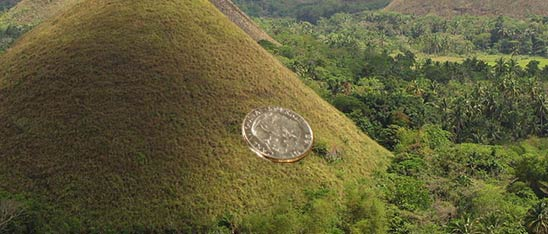# Faster and faster we goA coin is constrained to move on the surface of a hill and slides from the top of a hill towards the bottom. The height y of the hill as a function of horizontal position is given by $y=1- \alpha x^2$, where y and x are both measured in meters and $\alpha=1~m^{-1}$. The coin has a mass of 10 g and is at the top of the hill at t=0 with an initial energy of 0.103 J (where we set the zero of potential energy at y=0). When the coin has reached x=1 m, what is the horizontal component of the coin's velocity in meters/second?

Details and assumptions

• You may neglect friction and air resistance.
• The acceleration of gravity is $-9.8~m/s^2$.
×